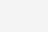# EXCEL条件格式区域固定不随插入删除而改变解决方法

1.1公式-名称管理器-编辑名称：例如：条件，引用位置设置公式：=OR(N(INDIRECT({“B”,”C”}&ROW()))>0)1.2开始-条件格式-编辑格式规则：使用公式确定要设置格式的单元格：输入  =条件，在设置行变色区域即可。2.1如果是列指定唯一值公式：公式-名称管理器-编辑名称：例如：借方 贷方，引用位置设置公式：例如：

```名称：借方，引用位置公式：=OR(N(INDIRECT({"E"}&ROW()))=1122)2.2开始-条件格式-编辑格式规则：使用公式确定要设置格式的单元格：输入  =借方  或者 =贷方，在设置行变色区域即可。应用于范围：=\$A:\$H3如果涉及文本表达，用公式管理器貌似行不通，直接在条件格式表达：

3.1满足A列不等于0

`=INDIRECT("A"&ROW())<>0`

3.2满足H列文本为上月结转

`=INDIRECT("H"&ROW())="上月结转"`

3.3同时满足两个条件：A列不等于0，H列文本为上月结转

```=(INDIRECT("A"&ROW())<>0)*(INDIRECT("H"&ROW())="上月结转")
3.4使用公式确定要设置格式的单元格公式，实现H列有以上上年结转、上月结转、本月合计、本年累计时自动实现填充格式如上图，具体设置如下：``````条件格式设置：

=OR(\$H1=INDIRECT("字段!A1:A4"))     应用于： =\$A:\$K

=OR(\$H1="本月合计",\$H1="本年累计")   应用于： =\$K:\$K```

(8)

6.5K

4.5K

6.6K

6.5K

5.4K